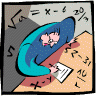## Mathematics, Department of## Dissertations, Theses, and Student Research Papers in Mathematics

David Pitts

3-2019

#### Comments

A DISSERTATION Presented to the Faculty of The Graduate College at the University of Nebraska In Partial Fulfillment of Requirements For the Degree of Doctor of Philosophy, Major: Mathematics, Under the Supervision of Professor David Pitts. Lincoln, Nebraska: March, 2019.

Copyright (c) 2019 Derek DeSantis

#### Abstract

Operator algebras generated by partial isometries and their adjoints form the basis for some of the most well studied classes of C*-algebras. Representations of such algebras encode the dynamics of orthonormal sets in a Hilbert space.We instigate a research program on concrete operator algebras that model the dynamics of Hilbert space frames.

The primary object of this thesis is the norm-closed operator algebra generated by a left invertible $T$ together with its Moore-Penrose inverse $T^\dagger$. We denote this algebra by $\mathfrac{A}_T$. In the isometric case, $T^\dagger = T^*$ and $\mathfrac{A}_T$ is a representation of the Toeplitz algebra. Of particular interest is the case when $T$ satisfies a non-degeneracy condition called analytic. We show that $T$ is analytic if and only if $T^*$ is Cowen-Douglas. When $T$ is analytic with Fredholm index $-1$, the algebra $\mathfrac{A}_T$ contains the compact operators, and any two such algebras are boundedly isomorphic if and only if they are similar.

Adviser: David Pitts

COinS